PSABM5X7 - Practice---Acc7.5 Lesson 15 Solving More Systems (6.EE.B.5, 8.EE.C.8)

Solve: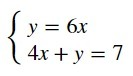(x,y)

with no spaces.

Solve: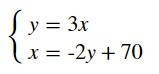(x,y)

with no spaces

Which equation, together with -1.53, makes a system with one solution?

A.  y = -1.5x + 6

B.  y = -1.5x

C.  2y = -3x + 6

D.  2y + 3x = 6

E.  y = -2x + 3

Select one:
Part A)

The system − 643− 184 has no solution.

Change one constant or coefficient to make a new system with one solution.

Part B)

Change one constant or coefficient to make a new system with an infinite number of solutions.

Part A)

Match each graph to its equation.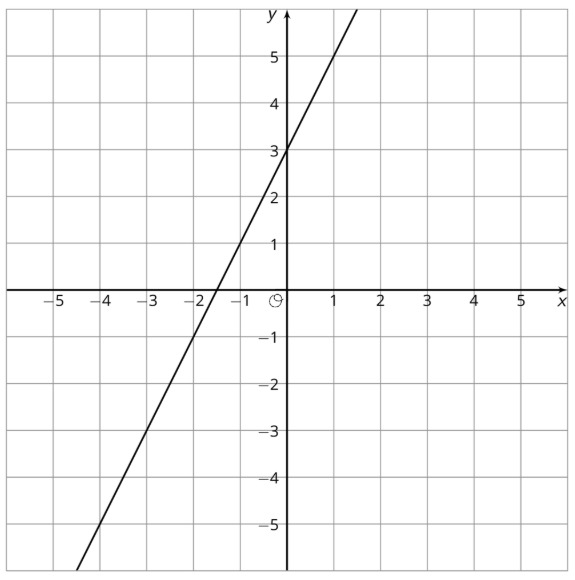Select one:
Part B)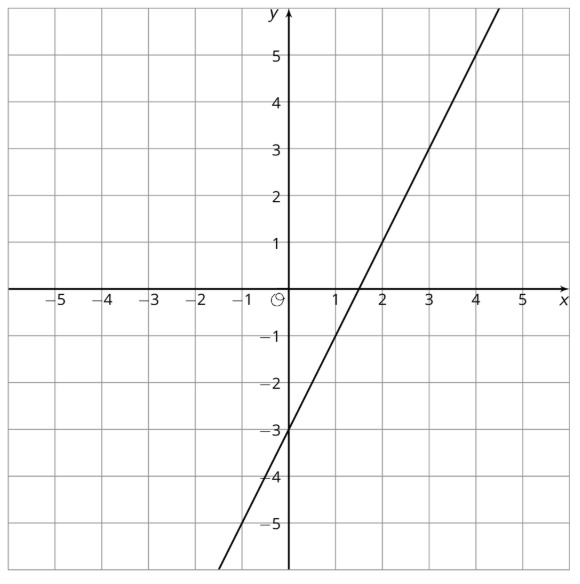Select one:
Part C)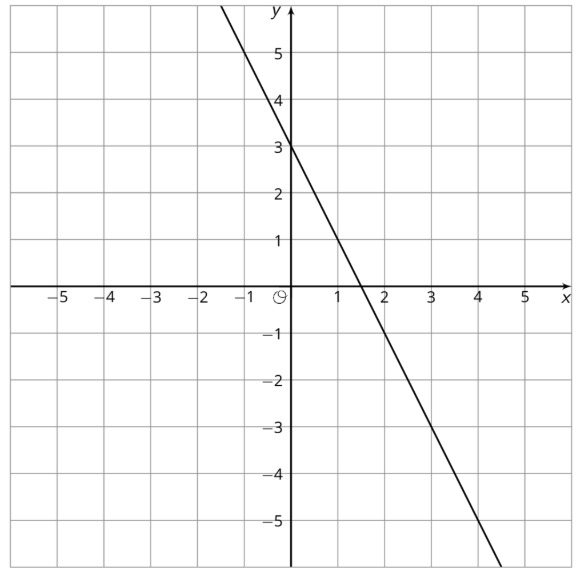Select one:
Part D)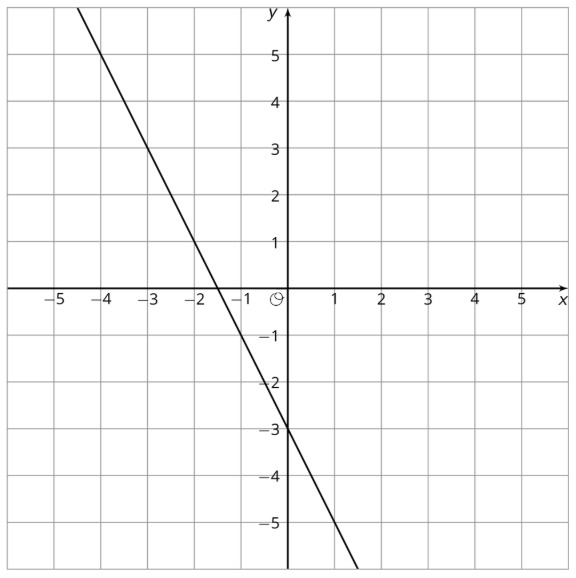Select one:

Here are two points: (-3,4)(1,7). What is the slope of the line between them?